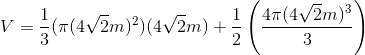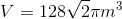# High School Math : How to find the volume of a polyhedron

## Example Questions

### Example Question #1 : How To Find The Volume Of A Polyhedron

Find the volume of the following half cylinder.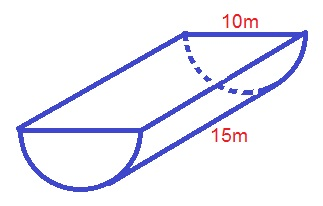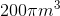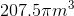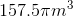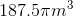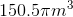Explanation:

The formula for the volume of a half-cylinder is: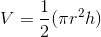whereis the radius of the base andis the length of the height.

Plugging in our values, we get: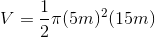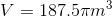### Example Question #2 : How To Find The Volume Of A Polyhedron

Find the volume of the following polyhedron.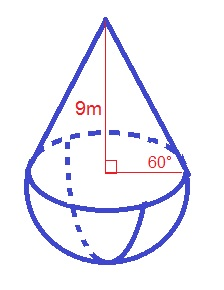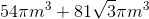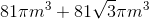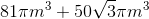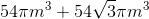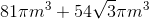Explanation:

The formula for the volume of the polyhedron is: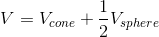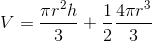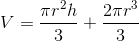Whereis the radius of the cone,is the height of the cone, andis the radius of the sphere.

Use the formula for a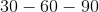triangle to find the length of the radius: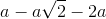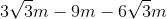Plugging in our values, we get: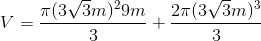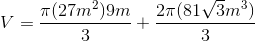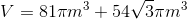### Example Question #3 : How To Find The Volume Of A Polyhedron

Find the volume of the following polyhedron.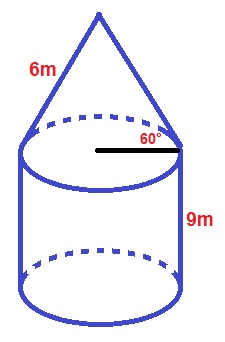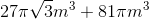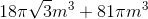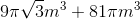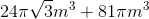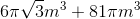Explanation:

The formula for the volume of the polyhedron is: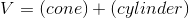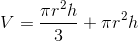whereis the radius of the cone,is the height of the cone,is the radius of the cylinder, andis the height of the cylinder.

Use the formula for atriangle to find the length of the radius and height of the cone: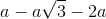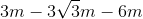Plugging in our values, we get: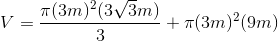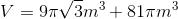### Example Question #8 : Other Polyhedrons

Find the volume of the following polyhedron.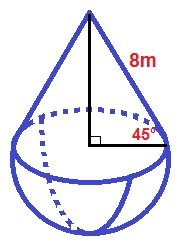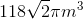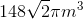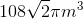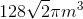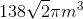Explanation:

The formula for the volume of the polyhedron is: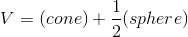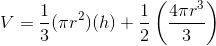whereis the radius of the polyhedron andis the height of the cone.

Use the formula for a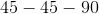triangle to find the length of the radius and height: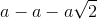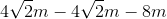Plugging in our values, we get: